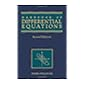Normal view MARC view ISBD view

# Handbook of differential equations.

 No cover image available No cover image available515.4 SZA Differential inequalities. 515.4 VER Nonlinear differential equations and dynamical systems. 515.4 ZWI Handbook of differential equations. Second edition. 515.4 ZWI Handbook of differential equations. 515.41 ARN Ordinary differential equations. 515.41 ARN Geometrical methods in the theory of ordinary differential equations. 515.41 ARN Geometrical methods in the theory of ordinary differential equations. Second edition. Translated by Joseph Szucs. English translation edited by Mark Levi. Second edition.